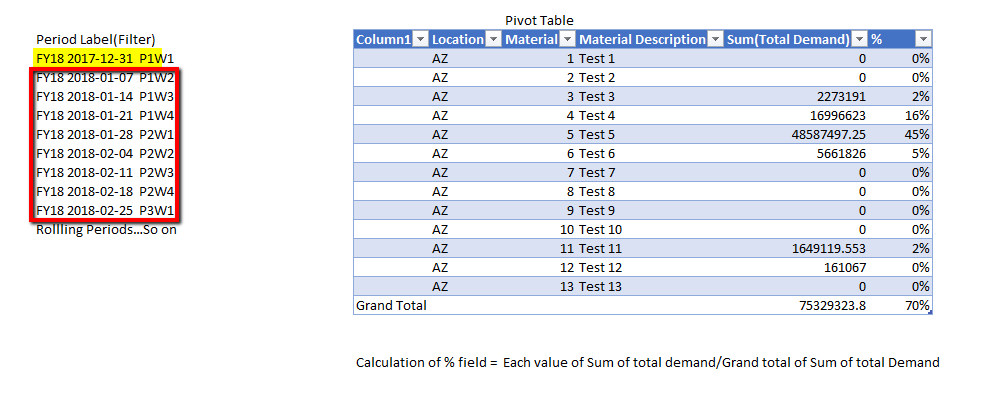# New to Qlik Sense

If you’re new to Qlik Sense, start with this Discussion Board and get up-to-speed quickly.

Announcements
Become an analytics expert with Qlik's new 15 week course: Applied Data Analytics using Qlik Sense. READ MORE
cancel
Showing results for
Did you mean:Contributor III

## Calculation of Rolling period by 8 weeks to get the sum of appropriate measure

Hi Everyone,

I want to Calculate the the Sum of total Demand by different dimensions(Material/Location/Material Desc ..etc) using the Period Label Filter.

Here I have to use Period Label Filter as my selection, If i have selected the current period as FY18 2017-12-31 P1W1 then the sum of total demand by each material should give me the Total 9 weeks demand (i.e, Current week (Highlighted Yellow)+ next 8 weeks(Highlighted Red) =9 weeks Total Demand by material). Period Label is Rolling with total of 52 weeks.

For Calculating the % field ,formula used  =  Each value of Sum of total demand/Grand total of Sum of total Demand

Any suggestion/help is appreciated.Thanks.

Labels (1)
• ### Calculation of Rolling period by 8 weeks to get the sum of appropriate measure